# Why Is Binary Used in Electronics and Computers?

Eugene is a qualified control/instrumentation engineer Bsc (Eng) and has worked as a developer of electronics & software for SCADA systems.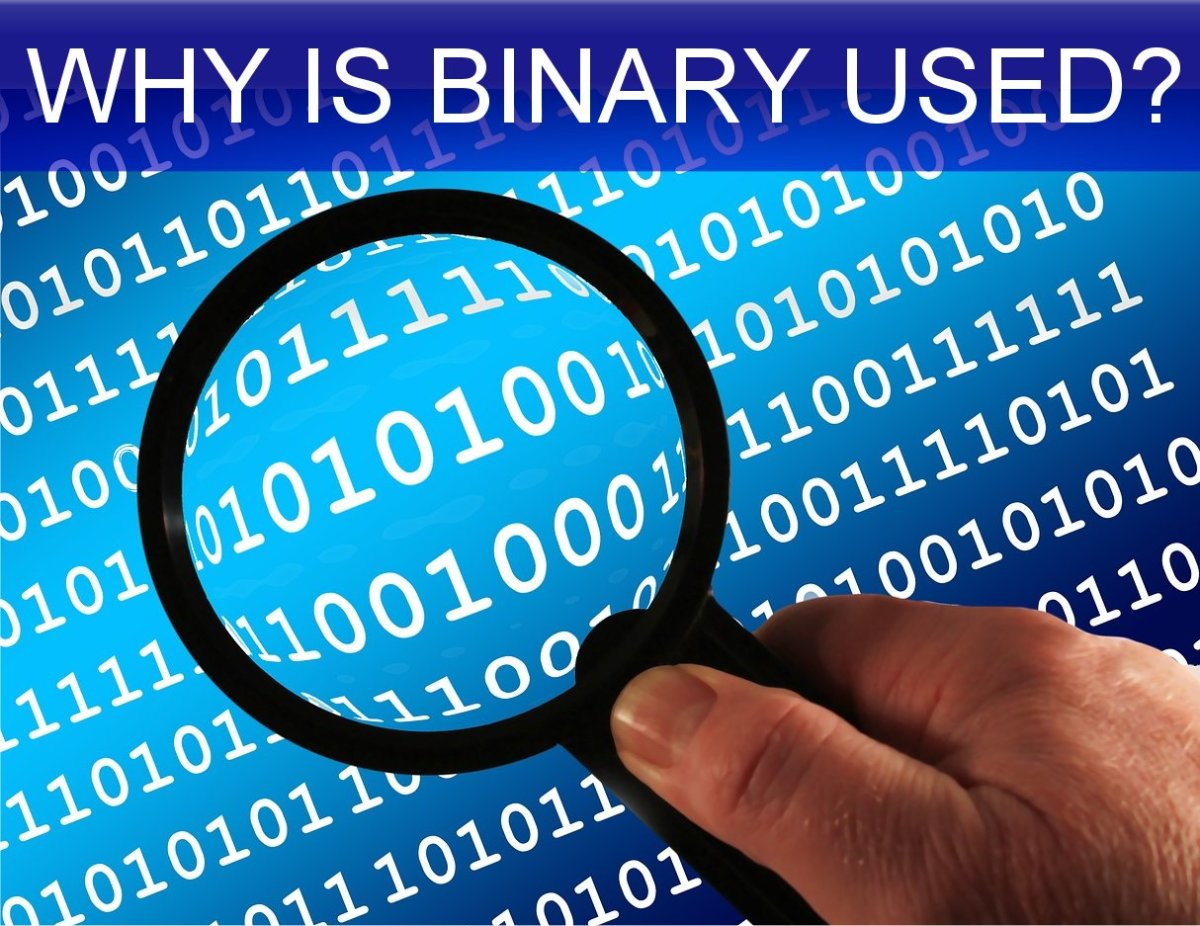Why is binary used in electronics?Geralt via Pixabay.com

## The Binary System

The binary numbering system is the basis for storage, transfer and manipulation of data in computer systems and digital electronic devices. This system uses base 2 rather than base 10, which is what we are familiar with for counting in everyday life. By the end of this easy-to-understand article, you'll have a grasp of why binary is used in computers and electronics.

## What Is Decimal and Why Do We Use It?

The decimal, base 10 or denary numbering system is what we are familiar with in everyday life. It uses 10 symbols or numerals.
To count we go

0, 1, 2, 3, 4, 5, 6, 7, 8, 9 . . .

...but there's no numeral for the next number, the integer value we interpret as "ten". Ten is therefore represented by two digits: the numeral 1 followed by 0 so ten is represented as "10", which really means "one ten and no units". Similarly, one hundred is represented by three digits: 1, 0 and 0; i.e., one hundred, no tens and no units".

Basically numbers are represented by a series of numerals in the units, tens, hundreds, thousands place etc. For instance, 134 means one hundred, three tens and four units. The decimal system probably arose because we have 10 fingers on our hands, which could be used for counting.

## What Is Binary and How Does It Work?

The binary system used by computers is based on two numerals: 0 and 1. So you count

0, 1

....but there's no numeral for 2. So 2 is represented by 10 or "one 2 and no units". In the same way that there is a units, tens, hundreds, thousands place in the decimal system, in the binary system there is a units, twos, fours, eights, sixteens place etc. in the binary system. So the binary and decimal equivalents are as below:

• 00000000 = 0
• 00000001 = 1
• 00000010 = 2
• 00000011 = 3
• 00000100 = 4
• 00000101 = 5
• 00000110 = 6
• 00000111 = 7 (and so on)

## Counting in Binary and Decimal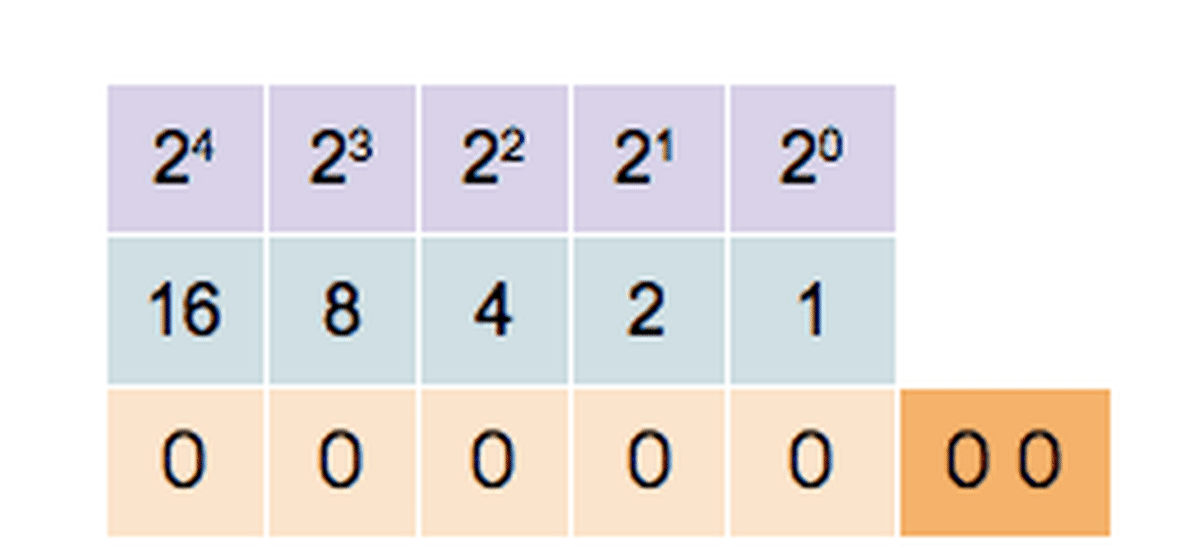Counting in binary from 0 to 11111 = 31 decimalEphert, CC by SA 4.0 via Wikimedia Commons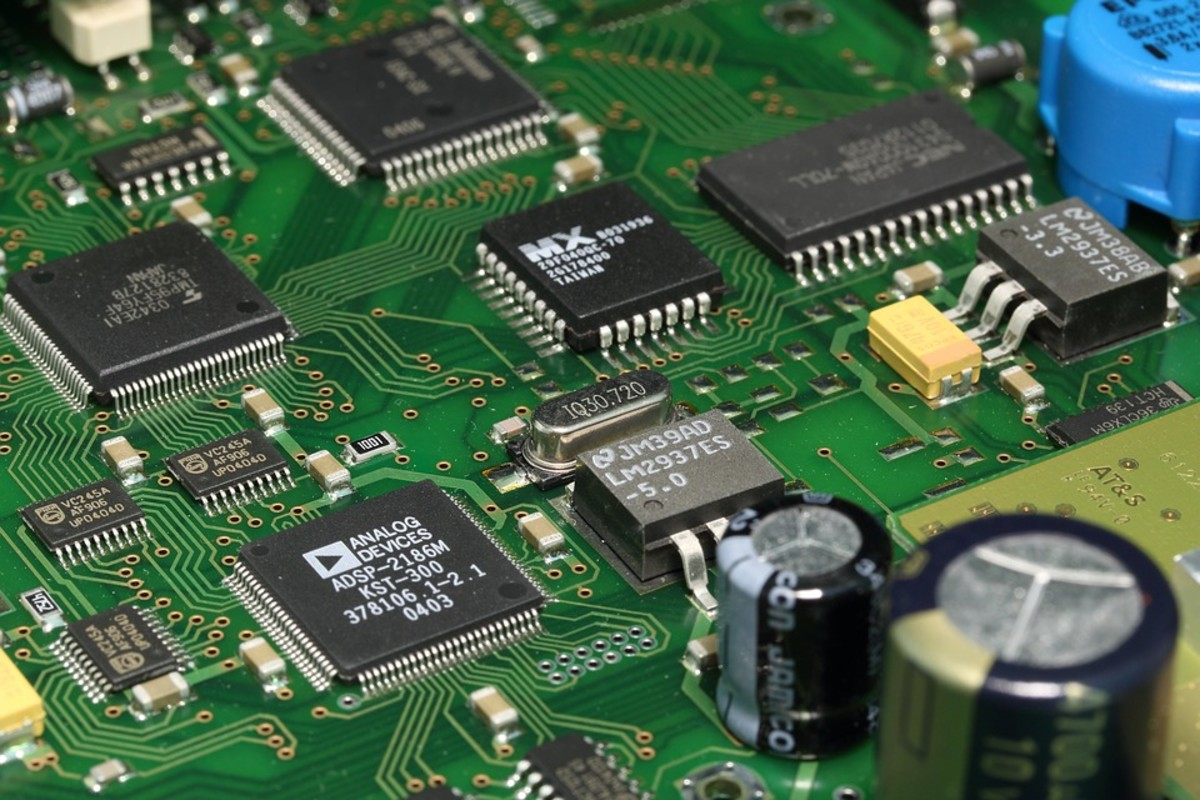A printed circuit board (PCB) with digital integrated circuits (ICs or "chips")Olafpictures, public domain image via Pixabay.com

## How Is Binary Used in Digital Computers and Electronic Devices?

Numbers can be encoded in binary format and stored using switches. The digital technology which uses this system could be a computer, calculator, digital TV decoder box, cell phone, burglar alarm, watch etc. Values are stored in binary format in memory, which is basically a bunch of electronic on/off switches.

Imagine if you had a bank of 8 rocker switches just like in the image below. Each switch could represent 1 or 0 depending on whether it is turned on or off. So you think of a number and set the switches on or off to "store" the binary value of this number. If someone else then looked at the switches, they could "read" the number.

## 8 Bit "Memory" Made From a Gang of Rocker Switches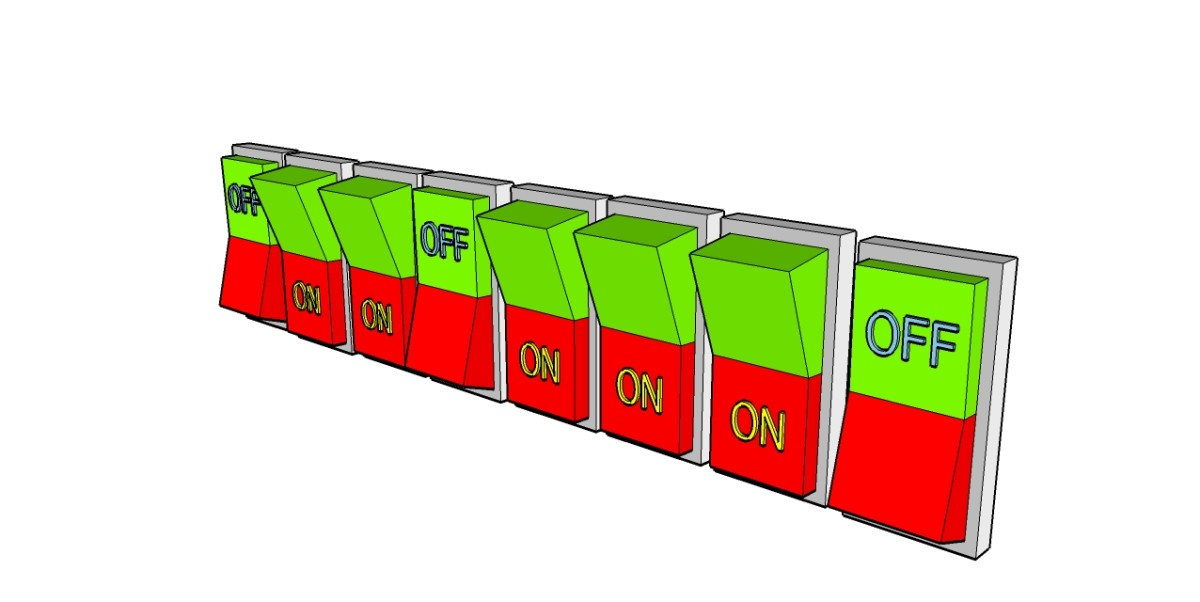Conceptual idea of how the state of a bank of eight switches allows the "storage" of 2 to the power of 8 = 256 possible numbers© Eugene Brennan

## How Does a Computer Implement Switches

So how does a computer store binary numbers? Obviously banks of rocker switches would be ridiculously impractical (although a similar technique was used in early computers when programming) In a computer, switches are implemented using microminiature transistors.

Scroll to Continue

The smallest memory configuration is the bit, which can be implemented with one switch. If 8 switches are added together you get a byte. The digital hardware is able to set the switches on and off (i.e. write data to a byte) and also read the state of the switches. In the conceptual image of rocker switches we saw above, there are 8 switches and 28 = 256 permutations or arrangements depending on whether a switch is on or off. If on represents 1 and off represents 0 for each switch, the group of switches can represent any of the following values.

• 00000000 0 decimal
• 00000001 1 decimal
• 00000010 2 decimal
• 00000011 3 decimal
• 00000100 4 decimal
• ...
• 11111110 254 decimal
• 11111111 255 decimal

In an electronic device or computer, because of micro-miniaturization, billions of switches can be incorporated onto integrated circuits (IC), potentially allowing the storage and manipulation of huge amounts of information.

BinaryDecimal

0

0

1

1

10

2

11

3

100

4

101

5

110

6

111

7

1000

8

1001

9

1010

10

1011

11

1100

12

1101

13

1110

14

1111

15

10000

16

## Representing Non-Integer Values in Computer Systems

Integers can be stored and processed directly as their binary equivalents in computer systems; however, this isn't the case with other data. A machine such as a computer, digital camera, scanner etc cannot directly store decimals, non-numerical (text, image, video) or analog measurement data from the real world directly. This type of data could be:

• Person's name or address
• Temperature measured in a room
• Image from a digital camera or scanner.
• Audio
• Video
• Decimal number

## Representing Data in Floating Point Format

Decimal numbers are represented in computer systems using a system known as floating point. A decimal number can be represented approximately, to a certain level of accuracy by an integer significand multiplied by a base, raised to the power of an integer exponent.

## Processing and Storage of Analog Data

A voltage level from a temperature sensor is an analog signal and has to be converted to a binary number by a device called an analog to digital converter (ADC). These devices can have various resolutions and for a 16 bit converter, the signal level is represented by a number from 0 to 216 = 65535. ADCs are also used in image scanners, digital cameras, in electronic equipment used to record sound and video and basically any digital device that takes input from a sensor. An ADC converts a real world analog signal into data that can be stored in memory. Images created in a CAD drawing package are also broken down into individual pixels and a byte of data is used for the red, green and blue intensity levels of each pixel.
What's the Difference Between Analog and Digital? explains this in more detail.

## Analog and Digital Signals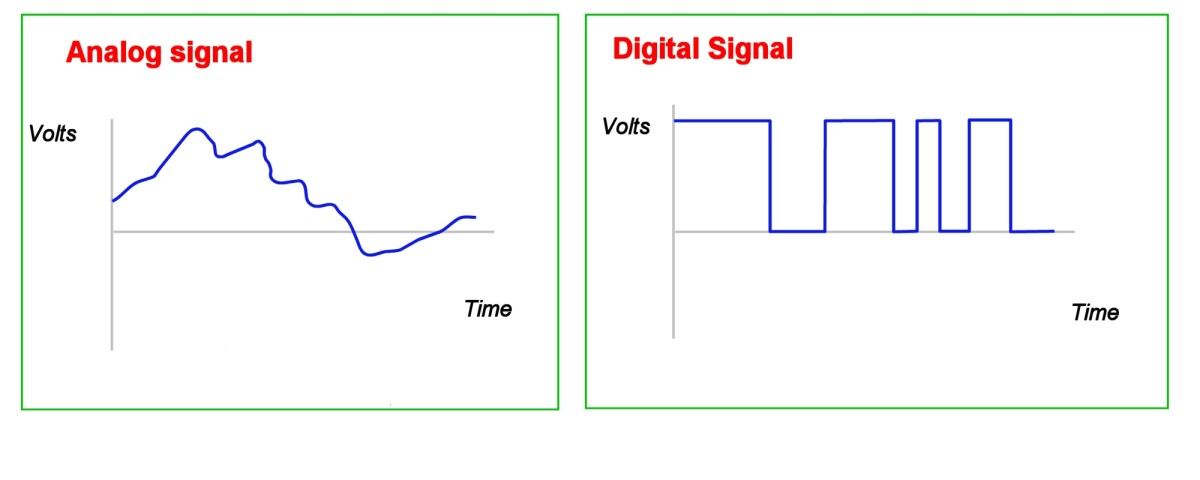Digital signals in electronic circuitry are either high or low, representing a "1" or "0"© Eugene Brennan

## Encoding Text Data as ASCII

Names, addresses or other text entered into a computer can't be stored directly in computer memory. Instead the text is broken down into individual letters, numerals and other non alphanumeric characters (e,g, &*£\$# etc) and a coding system called ASCII represents each character by a number from 0 to 127. This data is then stored in binary format as one or more bytes in memory, each byte being made up of individual bits, and each bit implemented using transistors.

## ASCII Table Hex, Binary and Decimal Values

Table showing ASCII characters with their hexadecimal, binary and decimal values. Hexadecimal or "hex" is a convenient way of representing a byte or word of data. Two characters can represent 1 byte of data.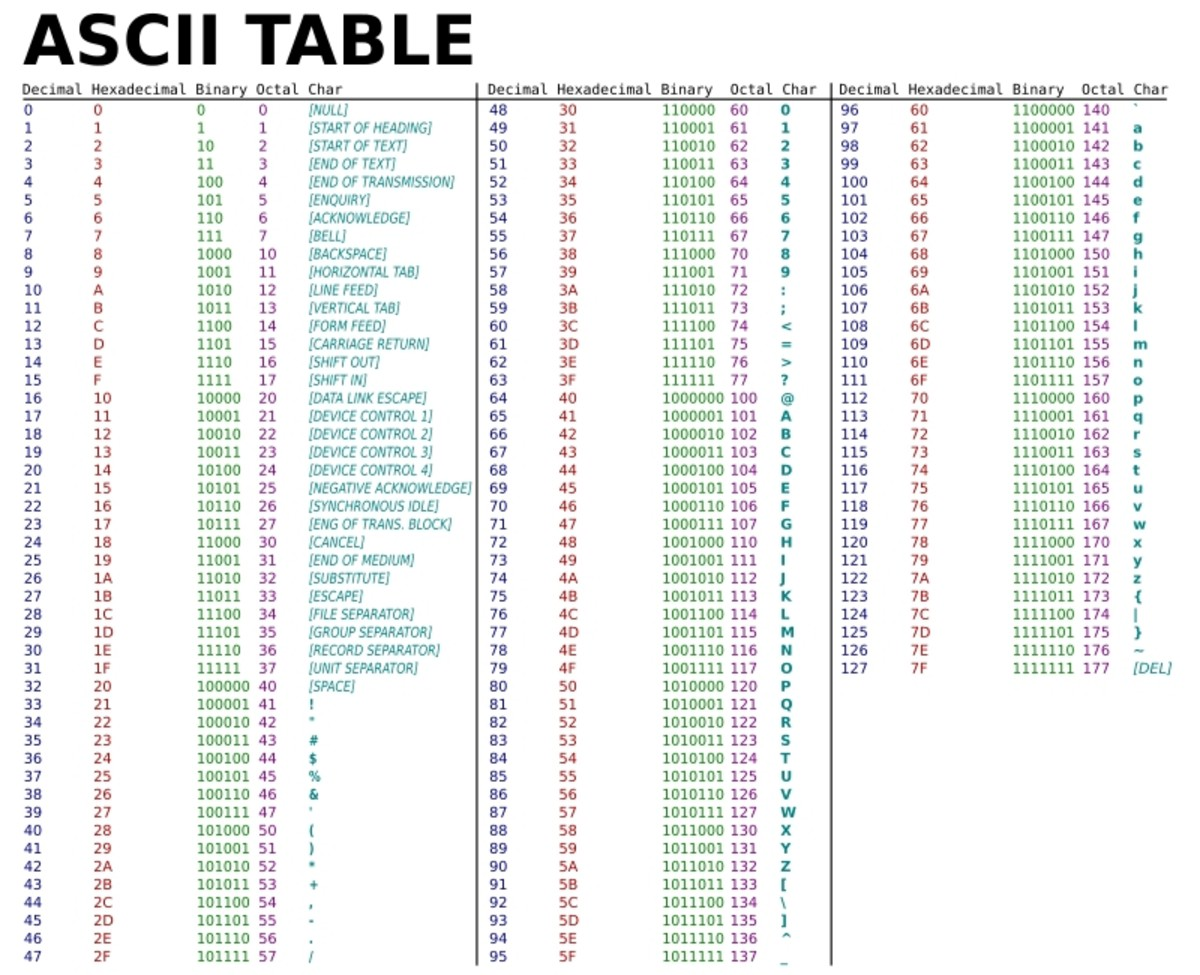ASCII code table. ASCII assigns a number from 0 to 127 to letters, numbers, non alphanumeric characters and control codesPublic domain image via Wikimedia Commons

## What Is Machine Code and Assembly Language?

Not only are values or data stored in memory, but also the instructions which tell the microprocessor what to do. These instructions are called machine code. When a software program is written in a high level language such as BASIC, Java or "C", another program called a compiler breaks the program down into a set of basic instructions called machine code. Each machine code number has a unique function which is understood by the microprocessor. At this low level, instructions are basic arithmetic functions such as add, subtract and multiply involving the contents of memory locations and registers (a cell which can have arithmetic operations carried out on it). A programmer can also write code in assembly language. This is a low level language comprising instructions known as mnemonics which are used to move data between registers and memory and perform arithmetic operations.

## How to Convert Decimal to Binary and Binary to Decimal

You can convert decimal to binary using the remainder method. See my guide for details:

How to Convert Decimal to Binary and Binary to Decimal

## George Boole and Boolean Algebra

Boolean algebra, developed by the British mathematician George Boole in the 19th century, is a branch of mathematics which deals with variables which can only have one of two states, true or false. In the 1930s Boole's work was discovered by the mathematician and engineer Claude Shannon, who realized it could be used to simplify the design of telephone switching circuits. These circuits originally used relays which could be either on or off, and the desired output state of the system, depending on the combination of states of the inputs, could be described by a Boolean algebraic expression. Boolean algebra rules could then be used to simplify the expression, resulting in a reduction of the number of relays required to implement a switching circuit. Eventually Boolean algebra was applied to the design of digital electronic circuitry as we will see below.

## Digital Logic Gates: AND, OR and NOT

A digital state, ie high/low or 1/0 can be stored in a one-bit cell in memory, but what if that data has to be processed? The most basic processing element in a digital electronic circuit or computer is a gate. A gate takes one or more digital signals and generates an output. There are three types of gates: AND, OR and NOT (INVERT). In their simplest form, small groups of gates are available on a single IC. However, a complex combinational logical function can be implemented using a Programmable Logic Array (PLA) and more sophisticated devices such as microprocessors are composed of millions of gates and memory storage cells.

• For an AND gate, the output is true or high only when both inputs are true.
• For an OR gate, the output is high if either or both inputs are true.
• For a NOT gate or inverter, the output is the opposite state to the input.

Boolean algebraic expressions can be used to express what the output signal of a circuit should be, depending on the combination of inputs. The main operations in Boolean algebra are and, or and not. During a design process, the required value of an output for all the various permutations of input states can be tabulated in a truth table. The value '1' in the truth table means an input/output is true or high. The value '0' means the input/output is false or low. Once a truth table is created, a Boolean expression can be written for the output, simplified and implemented using a collection of logic gates.

So a typical Boolean expression with three independent variables A, B and C and one dependent variable D would be:

Y = A.B + C

This is read as "Y = (A and B) or C"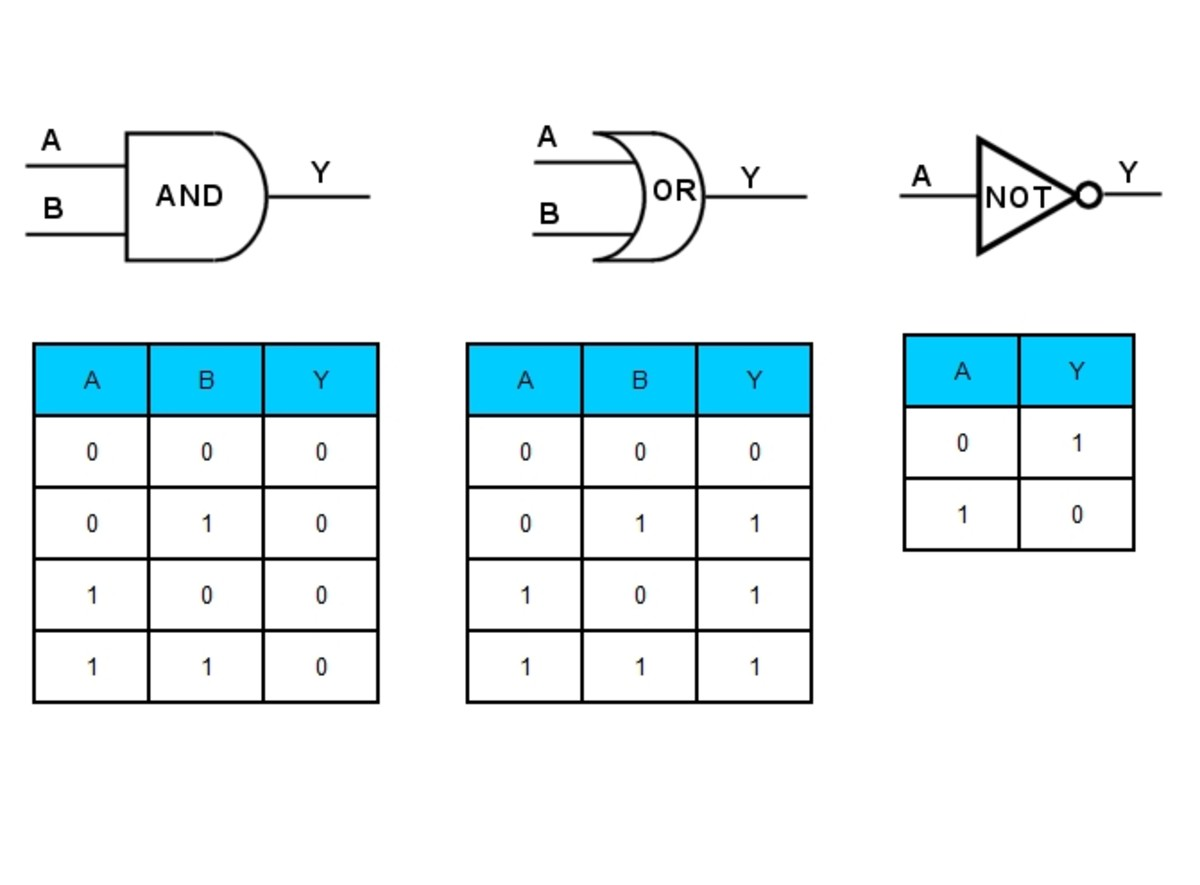Logic gates, AND, OR and NOT and their truth tables© Eugene Brennan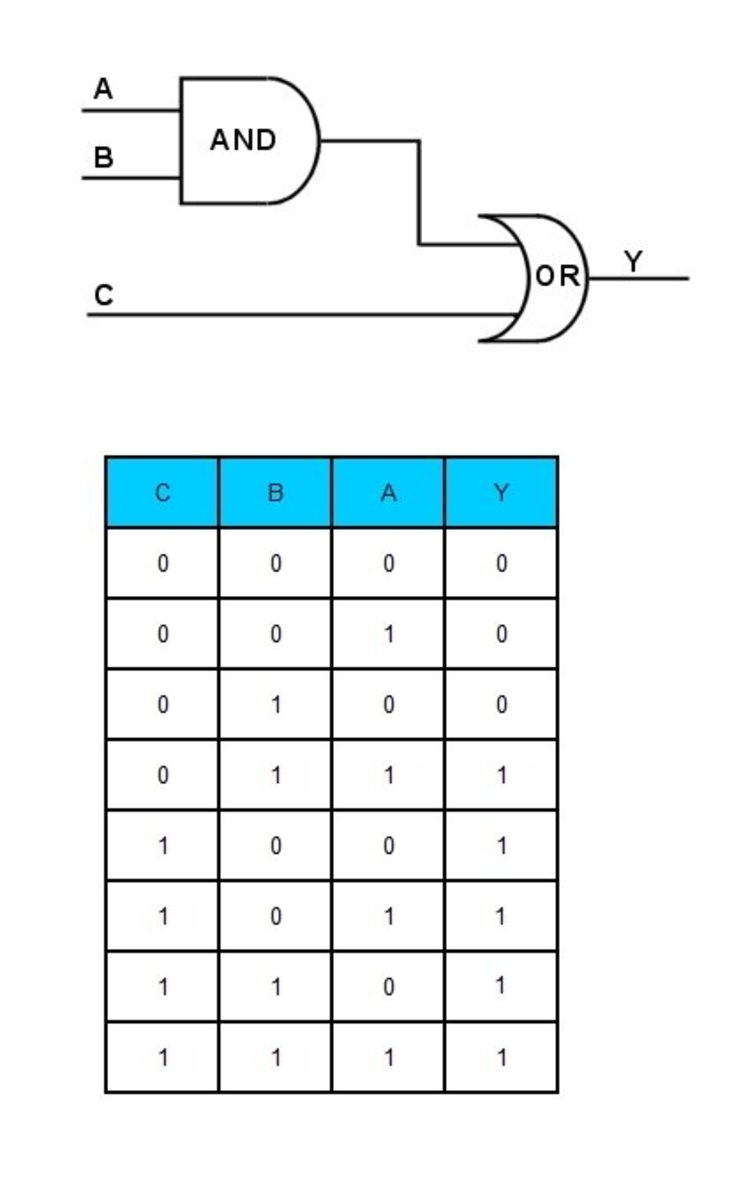Truth table for a simple digital circuit. Y = A.B + C© Eugene Brennan

This content is accurate and true to the best of the author’s knowledge and is not meant to substitute for formal and individualized advice from a qualified professional.

## Questions & Answers

Question: What is 16 in the octal number system?

Answer: If you mean 16 is an octal number, and you want to convert to decimal, the answer is 16 = 1 x 8 + 6 = 14 decimal.

If you mean how do I represent 16 decimal in the octal numbering system (base 8), the answer is 20 (2 in the "eights" place).

Question: What are the applications of a binary encoding system?

Answer: Binary encoding is a system of transmitting binary data over a link designed to handle text data. e.g. email.

You can read more about it here:

https://en.wikipedia.org/wiki/Binary-to-text_encod...

Question: Why can't we use -1 in digital electronics?

Answer: -1 or other negative numbers are normally implemented using two's complement. So to represent -1 in two's complement form, invert the bits and add 1:

So 1 is 001

Inverting the bits gives

110

111

Remember this is just a conventional way of representing a negative number in a digital system so that arithmetic can be done and work out ok.

So adding -1 and 1 gives -1 + 1 = 0

or in binary 111 + 001 = 1000.

Because only three bits are used, the 4th bit would be "unseen" by the digital system and the result in this example would be interpreted as 000 or zero.

You can more about two's compliment here:

https://en.wikipedia.org/wiki/Two%27s_complement

Question: Which characteristic of a transistor is useful for digital counting and manipulation, and why?

Answer: A transistor can behave as a controlled switch and form part of an elementary circuit element called a flip-flop. A flip-flop can store one bit of information, and in addition to other circuit elements, a higher level device called a binary counter can be implemented.

Question: What voltages are used for 1 and 0 in a digital circuit?

Answer: It depends on the technology. Sometimes a voltage close to zero is used for logic 0 and a higher voltage is used for logic 1. However in the case of some serial data transmission standards, a negative voltage represents logic 1 and a positive voltage represents logic 0. Several voltage levels are used by digital integrated circuits (chips), e.g. 5 v logic uses voltages lower than this for logic 1 and 3 volt logic uses even lower voltages. RS232 data transmission may use voltages close to +1 20 volts.

Question: What are high and low in a digital circuit?

Answer: "1" and "0" by convention represent "high" and "low" in a digital circuit.

© 2012 Eugene Brennan

Nima on June 15, 2018:

The article was really helpful for my studies.. You did it very well . Thank you very much for the information

Eugene Brennan (author) from Ireland on April 15, 2018:

Hi Chirayu,

In the time before sophisticated computer graphics and when displays could only show text, alphanumeric characters were displayed on screen using a chip called a character generator ROM (Read Only Memory). This converted ASCII bytes in memory into a rectangular matrix of dots representing the character that circuitry in the computer and monitor could display.

Here's some info on how Atari computers used to work.

https://www.atariarchives.org/cgp/Ch02_Sec04.php

Nowadays everything you see on screen is stored as a raster image in memory with three bytes for each pixel you see on screen. One byte is for red, one for blue and one for green. Depending on the value of the three bytes, you can get 16 million different shades of colours.

Have a look at this tutorial which gives more detail.

http://graphicdesign.spokanefalls.edu/tutorials/te...

Chirayu on April 15, 2018:

Hello sir

I have a question ....

How computer imagine the design and shape of the binary code which we are writing ....

For example :- I right eight in binary of so how computer imagine and design the shape of eight on the screen

Kefas on January 03, 2018:

Great article, keep up with the brilliant work.

Kiran on July 11, 2017:

U guys made my problem easy now I m topper in electronics first I got 60% now I am getting 99%

Robert Sacchi on March 20, 2017:

It is a very good article that explains things very well. There is a simpler albiet less refined unrefined method of conversion. Decimal 27 to binary:

32 16 8 4 2 1

0 1 1 0 1 1 = 16+8+2+1 = 27

Binary 1011010 to decimal:

64 32 16 8 4 2 1

1 0 1 1 0 1 0 = 64+16+8+2 = 90 Decimal

5A Hexidecimal = 132 Octal

Crude but effective.

Dan Harmon from Boise, Idaho on August 05, 2012:

You explained this very well. Many years ago I experimented on programming in machine language - what a hassle! It didn't take long to move to something higher.